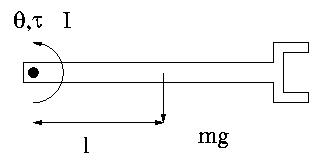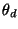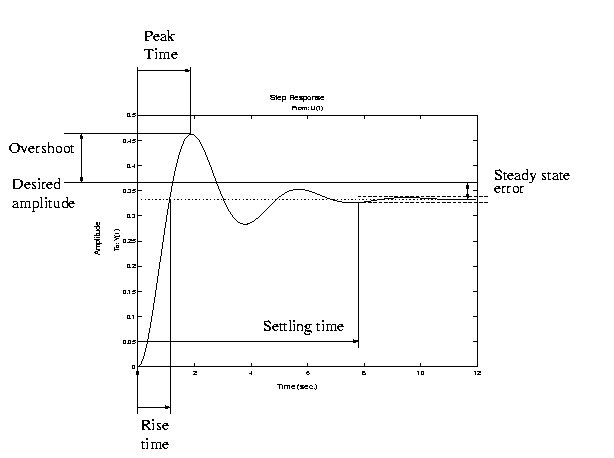### AME 301: Differential Equations, Vibrations and Control Homework 7

 B. GoodwineJ. Lucey Fall, 2003 Issued: October 20, 2003 Due: October 28, 2003 (US)23 October 2003 (UK)

### Introduction

The purpose of this homework is twofold. First, it is designed for you to develop intuition regarding the effects of changes of controller gains for PID control. Second, it will serve as a brief introduction to numerical techniques for solving differential equations, which is a subject we will study in detail near the end of the semester. The term numerical simulation simply means using a computer to solve the differential equation rather than using an analytical method. You were introduced to this in EG 111 or 112 and are probably familiar with the Euler method. Regardless, sample code is provided on the course web page to help you write your program.

Consider the robot arm illustrated in the following figure, with a mass m and moment of inertia I.1.
Derive the equation of motion for the arm and linearize the equation about(we did this in class).
2.
Via numerical simulation of the linearized equations, (pick your own values for I, m, l and, and use any programming language and/or environment you want) verify the following rules of thumb'' for PID control in light of the definitions of rise time, peak time, overshoot, settling time and steady state error from the following figure.Submit plots indicating the response of the system as well as the gain values used.
(a)
For proportional control, i.e., kp > 0, kd = 0 and kI=0, the solutions are oscillatory, and increasing kp increases the frequency of oscillation (which decreases the rise time and peak time) but decreases the mean steady state error. The settling time is infinite.
(b)
If derivative control is added to the proportional controller, i.e., kp > 0, kd > 0 and kI=0, then
i.
for small kd the solutions are decaying oscillations;
ii.
increasing kd decreases the settling time;
iii.
increasing kd sufficiently eliminates the oscillatory behavior completely, resulting in an solution which exponentially decays to the final, steady state value;
iv.
increasing kp decreases the final steady state error;
v.
increasing kp decreases the rise time.
(c)